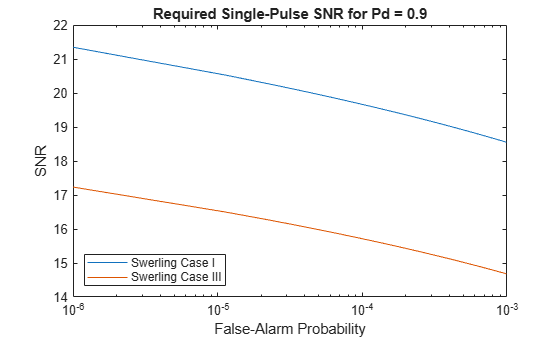# shnidman

Required SNR using Shnidman’s equation

## Syntax

``SNR = shnidman(Pd,Pfa)``
``SNR = shnidman(Pd,Pfa,N)``
``SNR = shnidman(Pd,Pfa,N,Sw)``

## Description

example

````SNR = shnidman(Pd,Pfa)` returns the required signal-to-noise ratio in decibels for the specified detection and false-alarm probabilities using Shnidman's equation. The SNR is determined for a single pulse and a Swerling Case Number of 0, a nonfluctuating target.```

example

````SNR = shnidman(Pd,Pfa,N)` returns the required SNR for a nonfluctuating target based on the noncoherent integration of `N` pulses.```

example

````SNR = shnidman(Pd,Pfa,N,Sw)` returns the required SNR for the Swerling case number `Sw`. ```

## Examples

collapse all

Find and compare the required single-pulse SNR for Swerling cases I and III. The Swerling case I has no dominant scatterer while the Swerling case III has a dominant scatterer.

Specify the false-alarm and detection probabilities.

```pfa = 1e-6:1e-5:.001; Pd = 0.9;```

Allocate arrays for plotting.

```SNR_Sw1 = zeros(1,length(pfa)); SNR_Sw3 = zeros(1,length(pfa));```

Loop over PFAs for both scatterer cases.

```for j=1:length(pfa) SNR_Sw1(j) = shnidman(Pd,pfa(j),1,1); SNR_Sw3(j) = shnidman(Pd,pfa(j),1,3); end```

Plot the SNR vs PFA.

```semilogx(pfa,SNR_Sw1) hold on semilogx(pfa,SNR_Sw3) hold off xlabel("False-Alarm Probability") ylabel("SNR") title("Required Single-Pulse SNR for Pd = "+Pd) legend("Swerling Case "+["I" "III"],Location="southwest")```The presence of a dominant scatterer reduces the required SNR for the specified detection and false-alarm probabilities.

## Input Arguments

collapse all

Probability of detection, specified as a positive scalar.

Data Types: `double`

Probability of false alarm, specified as a positive scalar.

Data Types: `double`

Number of pulses for noncoherent integration, specified as a positive scalar.

Data Types: `double`

Swerling case number, specified as `0`, `1`, `2`, `3`, or `4`. For more information, see Swerling Case Number

Data Types: `double`

collapse all

### Shnidman's Equation

Shnidman's equation is a series of equations that yield an estimate of the SNR required for a specified false-alarm and detection probability. Like Albersheim's equation, Shnidman's equation is applicable to a single pulse or the noncoherent integration of `N` pulses. Unlike Albersheim's equation, Shnidman's equation holds for square-law detectors and is applicable to fluctuating targets. An important parameter in Shnidman's equation is the Swerling case number.

### Swerling Case Number

The Swerling case numbers characterize the detection problem for fluctuating pulses in terms of:

• A decorrelation model for the received pulses

• The distribution of scatterers affecting the probability density function (PDF) of the target radar cross section (RCS).

The Swerling case numbers consider all combinations of two decorrelation models (scan-to-scan; pulse-to-pulse) and two RCS PDFs (based on the presence or absence of a dominant scatterer).

Swerling Case NumberDescription
0 (alternatively designated as 5)Nonfluctuating pulses.
IScan-to-scan decorrelation. Rayleigh/exponential PDF–A number of randomly distributed scatterers with no dominant scatterer.
IIPulse-to-pulse decorrelation. Rayleigh/exponential PDF– A number of randomly distributed scatterers with no dominant scatterer.
IIIScan-to-scan decorrelation. Chi-square PDF with 4 degrees of freedom. A number of scatterers with one dominant.
IVPulse-to-pulse decorrelation. Chi-square PDF with 4 degrees of freedom. A number of scatterers with one dominant.

## Version History

Introduced in R2011a